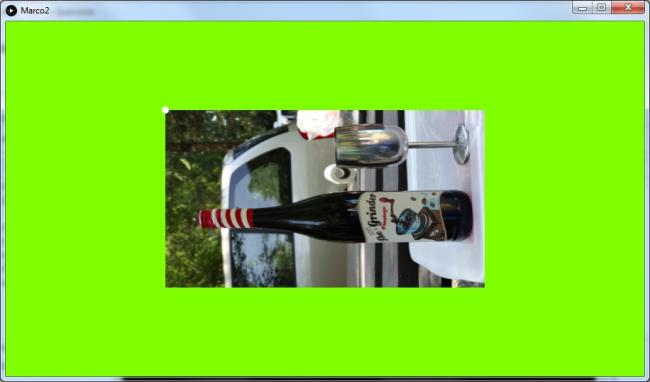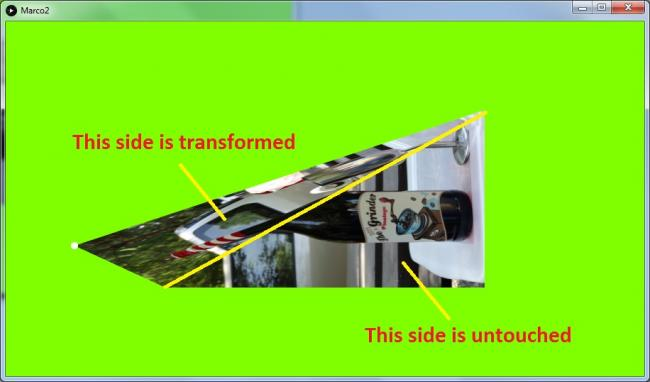#### Howdy, Stranger!

We are about to switch to a new forum software. Until then we have removed the registration on this forum.

# Strange behavior of Texture when transforming shape

edited July 2016

Hi,

(I'm pretty new to this "Fantastic" tool so please forgive if I'm just doing something obviously wrong)

I'm trying to transform a picture interactively using mouse control.

I've created some code (pasted at the end here) and all seems working fine other than the expected behavior of applied texture to shape.

If you try extending a corner of the shape (dragging any corner) the texture is transformed only in the triangle formed by the dragged corner and the two adjacent corners.

I'm attaching here 2 pictures showing the behavior for clarityHere's my code

``````class Quadro {
float [][] vertices;
int i;
int indexActive = -1;
PImage img;

Quadro () {
vertices = new float;
}

void setSize() {
vertices = width * 3 / 12;
vertices = height * 3 / 12;

vertices = width * 9 / 12;
vertices = height * 3 / 12;

vertices = width * 9 / 12;
vertices = height * 9 / 12;

vertices = width * 3 / 12;
vertices = height * 9 / 12;
}

void checkActiveVetices() {
float oldDistance = 100000000;
float distance;

for (i = 0; i < 4; i++) {
distance = dist(mouseX, mouseY, vertices[i], vertices[i]);
if (distance < oldDistance) {
indexActive = i;
oldDistance = distance;
}
}
}

void setActiveVertice() {
vertices[indexActive] = mouseX;
vertices[indexActive] = mouseY;
}

void setTexture(PImage i) {
img = i;
}

void paint() {
background(128, 255, 0);
fill(255);
noStroke();
beginShape();
textureMode(NORMAL);
texture(img);
vertex(vertices, vertices, 0, 0);
vertex(vertices, vertices, 1, 0);
vertex(vertices, vertices, 1, 1);
vertex(vertices, vertices, 0, 1);
endShape();

if ( indexActive >= 0) {
ellipse(vertices[indexActive], vertices[indexActive], 10, 10);
}
fill(255);
}
}

// define global variables
Quadro q = new Quadro();
PImage img;

void setup() {
size(900, 500, P3D);
q.setTexture(loadImage("PleaseAddYourImageHere.JPG"));
q.setSize();
q.paint();
}

void draw() {
q.checkActiveVetices();
if (mousePressed && (mouseButton == LEFT)) {
q.setActiveVertice();
}

q.paint();
}
``````

How can I transform the picture in a more expected proportional mode?

Thanks, Marco

Tagged:

## Answers

• Know problem. Search for affine texturing.

Breaking the shape up into more pieces is the usual solution.

• Hi koogs,

I see

Thanks, Marco

Sign In or Register to comment.